# Mathematics - Online Test

Q1. A circular template has a radius of 10 cm. The measurement of radius has an approximate error of 0.02 cm. Then the percentage error in calculating area of this template is
Explaination / Solution: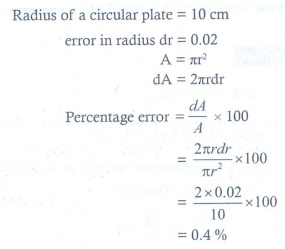Q2. 1/cos 80̊  - √3/sin 80̊ =
Explaination / Solution: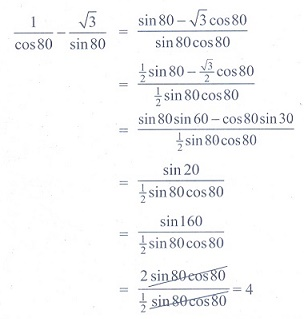Q3. The area of ellipse  is
Explaination / Solution:

Area of standard ellipse is given by :πab.

Q4. The distance of the point ( 3, 4, 5) from X- axis is
Explaination / Solution:

The distance of the point ( 3, 4, 5) from X- axis is

let L be the foot of perpendicular from the point ( 3, 4, 5) to X axis ,then coordinate of L will be (3,0,0)       [ because on X axis y and z coordinate are zero]

then distance of the point ( 3, 4, 5) from X- axis i.e. from L (3,0,0) is given by

Q5. If A and B are symmetric matrices of the same order, then
Explaination / Solution:

If A and B are symmetric matrices of the same order, then AB + BA is always a symmetric matrix.

Q6. If ∫ f (x)dx = g(x) + c, then ∫ f (x)g′(x)dx
Explaination / Solution: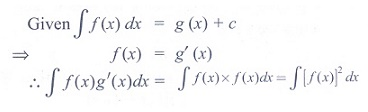Q7. in + in+1 + in+2 + in+3 is
Explaination / Solution:Q8. The value of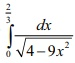is
Explaination / Solution: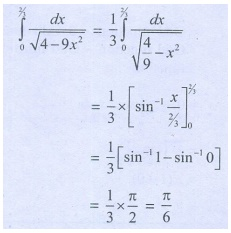Q9. The sum of the digits at the 10th place of all numbers formed with the help of 2, 4, 5, 7 taken all at a time is
Explaination / Solution: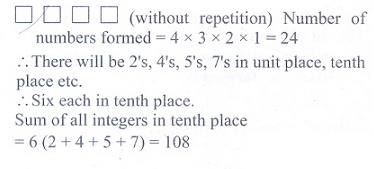Q10. The instantaneous rate of change at t = 1 for the function f (t) =  is
Explaination / Solution:

f(1)=e1+e1=0